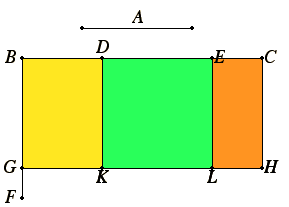Proposition 1

If there are two straight lines, and one of them is cut into any number of segments whatever, then the rectangle contained by the two straight lines equals the sum of the rectangles contained by the uncut straight line and each of the segments.

Let A and BC be two straight lines, and let BC be cut at random at the points D and E.I say that the rectangle A by BC equals the sum of the rectangle A by BD, the rectangle A by DE, and the rectangle A by EC.

Draw BF from B at right angles to BC. Make BG equal to A. Draw GH through G parallel to BC. Through D, E, and C draw DK, EL, and CH parallel to BG.

Then BH equals the sum of BK, DL, and EH.

Now BH is the rectangle A by BC, for it is contained by GB and BC, and BG equals A; BK is the rectangle A by BD, for it is contained by GB and BD, and BG equals A; and DL is the rectangle A by DE, for DK, that is BG, equals A. Similarly also EH is the rectangle A by EC.

Therefore the rectangle A by BC equals the sum of the rectangle A by BD, the rectangle A by DE, and the rectangle A by EC.

Therefore if there are two straight lines, and one of them is cut into any number of segments whatever, then the rectangle contained by the two straight lines equals the sum of the rectangles contained by the uncut straight line and each of the segments.

Q.E.D.

Guide

As described in II.Def.1, the phrase “the rectangle contained by the two straight lines” means any rectangle constructed with two sides equal to the two given sides. In some sense this is the product of the two lines. When the sides have names, such as A and BC, we will refer to that rectangle by “the rectangle A by BC” since that is a little clearer than Euclid’s terse “the rectangle A, BC.

In this proposition Euclid proves that if

BC = BD + DE + EC,
then
(A by BC) = (A by BD) + (A by DE) + (A by EC).

In modern algebraic notation this could be stated as follows: If y = y1 + y2 + ... + yn, then xy = x y1 + x y2 + ... + x yn. This can also be stated in a single equation as

x (y1 + y2 + ... + yn) = x y1 + x y2 + ... + x yn.

Here x and the various yi’s are all lines, and n is an arbitrary number. In modern terminology this identity is called the distributive law for multiplication over addition.

Use of this proposition

This proposition is not specifically invoked in the rest of the Elements. The next two propositions, however, are special cases of it, and they are each explicitly used once.